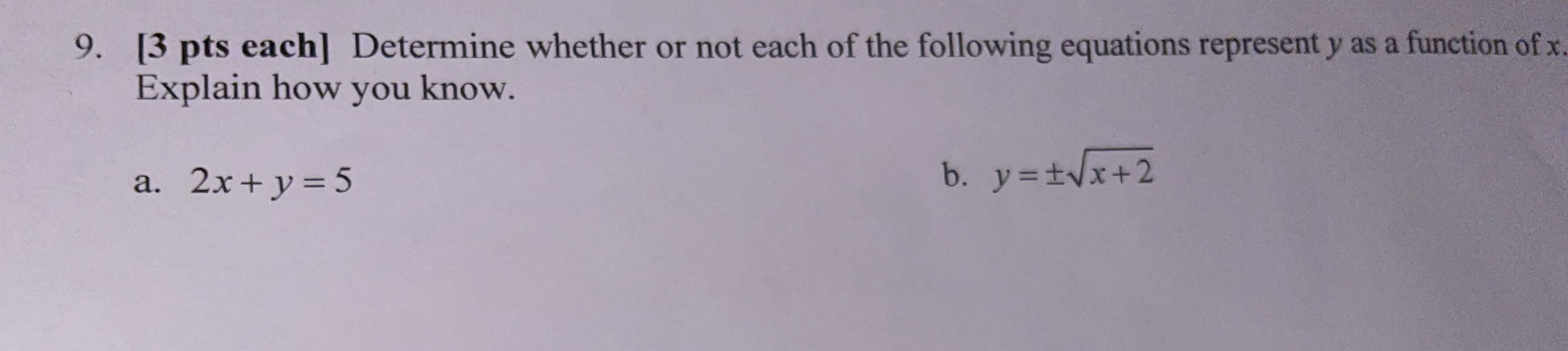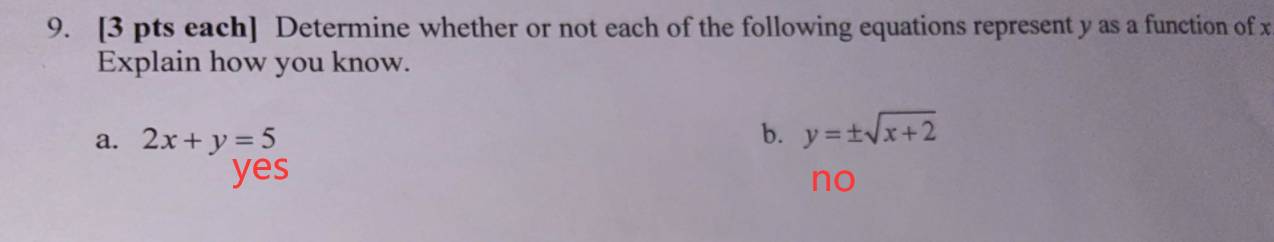### ¿Todavía tienes preguntas de matemáticas?

Pregunte a nuestros tutores expertos
Algebra
Pregunta

9.  Determine whether or not each of the following equations represent $$y$$ as a function of $$x$$ . Explain how you know.

a. $$2 x + y = 5$$ b. $$y = \pm \sqrt { x + 2 }$$